The naval hydrodynamics studies the resistance to the advancement of ships. Resistance to the advancement corresponds to the pushing force that will produce the propeller (see: Propeller calculation software). This resistance is composed of forces from several phenomena that are distinguished in the study but whose interactions are intertwined.

The results of calculations or measurements are usually given in the form of hydrodynamic coefficients Ch, relating the hydrodynamic resistance Rh, to the the weight of the volume of water displaced (Ch=Rh/Displacement)

We assume that the environment is quiet (no swell wind or current) and we limit our discussion by neglecting the resistance of superstructure (about 3% of Rh), to the study of ship resistance to the advancement bareboat, by dividing it into two parts :viscous resistance and wave resistance. (See Froude)
• viscous resistance (Rv), the viscous resistance coefficient (Cv) is related to Reynolds number (Re) and to roughness (K) relating hull length (L)
• Rv= Cv . (Re . K/L)

If the object moves near the surface, viscous resistance or drag is accompanied by a resistance due to the formation of surface waves:

• resistance of waves (Rw), the wave resistance coefficient (Cw) is related to Froude number(Fr)

• Rw= Cw.(Fr)

• Cw =Rw/(1/2)r.s.v²(r water density, S wet surface, V velocity.
the total hydrodynamic resistance coefficient (Ch=Rh/Displacement) for bareboat moving near the surface is defined as:
• Rv= Cv . (Re . K/L)

• Rw= Cw.(Fr)

• Ch= Cw.(Fr) + Cv.(Re.K/L)

Different types of approaches used to evaluate the hydrodynamic coefficient (Ch):

Despite advances in computer modeling, testing models are still essential.The effects of friction measured experimentally basins hulls, are themselves subject to scaling problems, solved by the laws of similarities(See: Froude)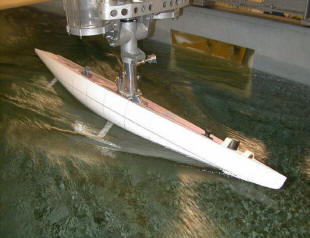testing model Towing tank

Another technique for evaluating hydrodynamic behavior is to compare measured data from a series of standard forms and extrapolate the consequences of changing forms.

 serie Taileur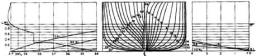serie NPL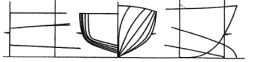serie Nordstrom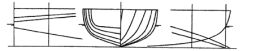63 serie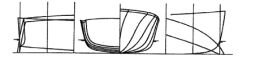serie SSPA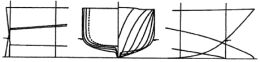64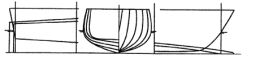• series taylor by Admiral Taylor Model Basin Experimental Ashington: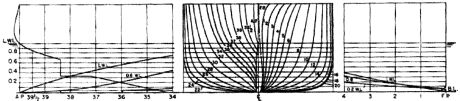The series of taylor is still regarded as the most comprehensive research into the effects on the effective power..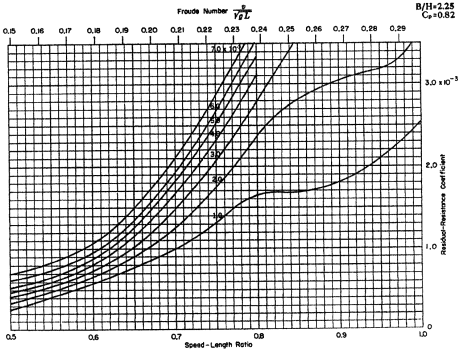.

top page

• In naval hydrodynamics, to independently evaluate the viscous resistance of the surface resistance, sometimes the method of dual models is used. It consists of a towing form consisting of a model hull of the ship and its symmetrical to the waterline. The measured drag is then halved. This experiment at sea is expensive and complex, it is carried in wind tunnel. For a simple and rapid assessment, it is possible to use the software mecaflux: The ovoid profile, whose drag (Cd) is already set in mecaflux, can be used to evaluate the viscous hydrodynamic resistance:

This viscous resistance is composed of the frictional resistance and resistance of shape. It is estimated that the roughness is very low (aerodynamic polished). If the roughness is higher, the frictional resistance due to roughness can be calculated with the detailed study of flat plate in Mecaflux standard (tab drag-lift / profiles with drag):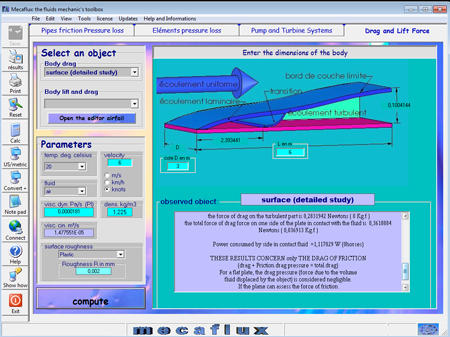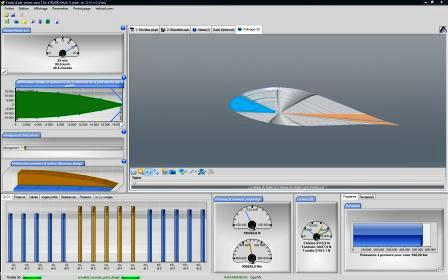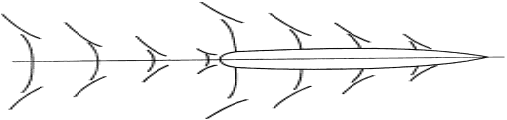A body which moves over a quiet water surface produces a wave system. This system is produced by the pressure field around the body, wave energy is given by the body. This transfer of energy from the body to the surrounding system produces wave resistance. (En bref le bateau fait des jolies vagues pour le Wake surf mais ça lui coute de l'énergie et c'est pour ça que c'est cher le Wake surf...)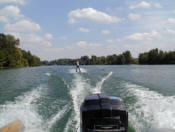There are two types of wave systems produced by hull:

1. A diverging wave system inclined relative to the axis of the vessel
2. A system of transverse waves perpendicular to the axis of the vessel.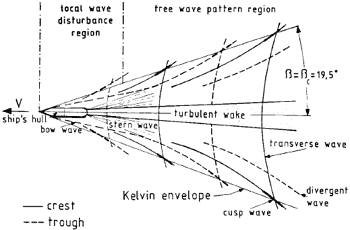The transversal waves is the main actor of the wave resistance at low Froude number.it is produced by the bow (front of ship) and stern (back of the ship). The interference between the wave systems generates peaks and troughs along the sides of the hull.

The total hydrodynamic resistance coefficient (Ch) is defined as:

Ch= Cw.(Fr) + Cv.(Re.K/L)

(Ch=Rh/Displacement)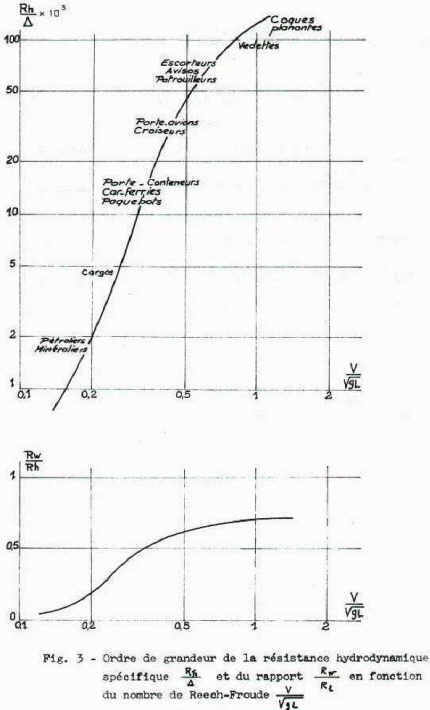top page